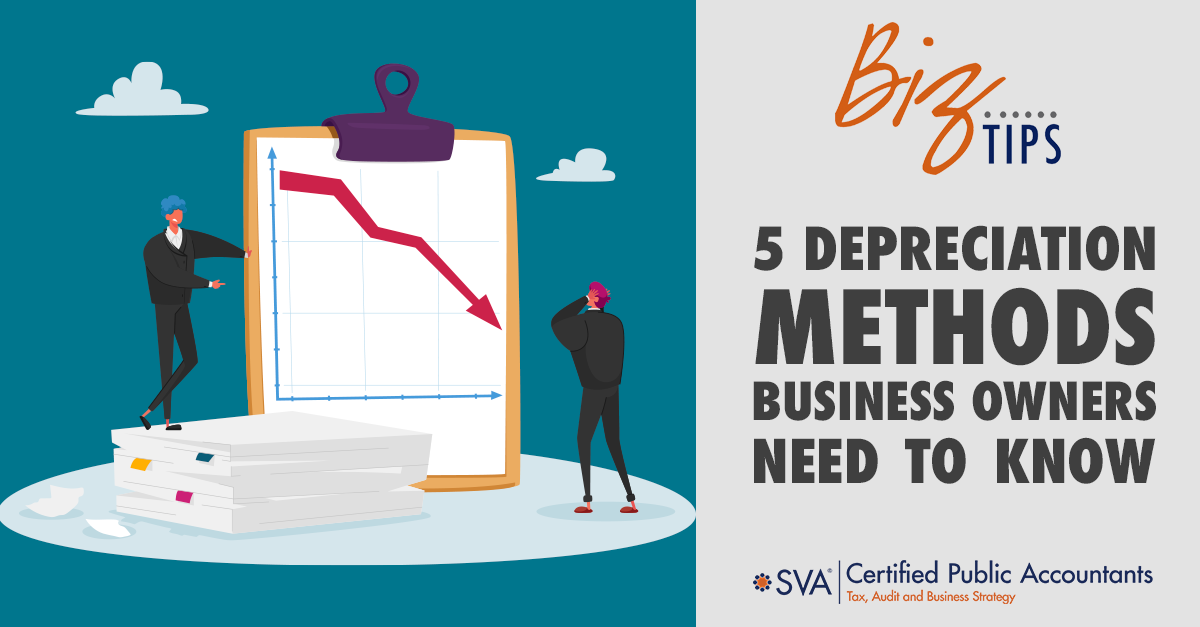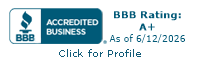# 5 Depreciation Methods Business Owners Need to Know

Depreciation of assets is an integral part of a company’s tax strategy which lowers the amount of earnings taxes are based on. This in turn reduces the amount of taxes owed.

Generally Accepted Accounting Principles (GAAP) give business owners the choice of 5 different methods of depreciation to use:

1. Straight Line
2. Declining Balance
3. Double Declining Balance
4. Sum of the Years' Digits
5. Units of Production

Each method calculates the rate of depreciation differently and some are better fits for different types of companies.

Some businesses choose one method for depreciating all their assets while some use two or more methods. The reason for using different methods could depend on the useful life of the asset or the company wanting larger deductions early.

## Methods of Depreciation

Before we look at each method more closely, let’s review the terms used in the formulas and what they mean.

Original Cost of the Asset

This is the initial cost that was paid for the asset.

Salvage Value

The estimated salvage price a business believes it would be able to get for the asset by selling it at the end of its useful life.

Useful Life of the Asset

The expected amount of time the asset will be of use to the company.

Current Book Value

The net value of an asset at the beginning of an accounting period. This is calculated by taking the cost of the asset and subtracting the accumulated depreciation.

Depreciation Rate

The rate (as a percentage) at which an asset is depreciated over its estimated lifespan. (Formula = 1/Useful Life of the Asset)

Remaining Lifespan

An estimate (in years) of an asset’s usefulness.

SYD (Sum of the Years' Digits)

The sum of the digits of an asset’s expected life. For example, if an asset is expected to last for 4 years, the SYD would be 10 (4 + 3 + 2 + 1 = 10).

Number of units an asset is expected to produce during its lifetime.

Number of units the asset produced during the current year.

### Straight Line Method

This is the simplest and most used depreciation method. It is best for smaller businesses that are looking for a simple way to calculate depreciation.

With the straight-line method, you are calculating a depreciation amount that is the same year after year for the life of the asset. This is what makes it the simplest method to use.

Example:

A company purchases a machine for \$10,000 that has a useful life of 5 years and a salvage value of \$2,000 at the end of its useful life. The company will use the straight-line depreciation method to depreciate the asset over its useful life. To calculate the depreciation expense for each year, the company will use the following formula:

#### Straight Line Depreciation Formula:

(Original Cost of the Asset - Salvage Value) / Estimated Useful Life of the Asset

In this case, the cost of the asset is \$10,000, the salvage value is \$2,000, and the useful life is 5 years. Plugging these values into the formula, we get:

Depreciation expense = (\$10,000 - \$2,000) / 5

Depreciation expense = \$1,600 per year

The company will record a depreciation expense of \$1,600 per year for the next 5 years. At the end of the useful life, the book value of the asset will be equal to the salvage value of \$2,000.

### Declining Balance Method

This method is best suited for companies that have assets that lose value faster in the early years. Technology (such as computers and cell phones) is an example of an asset that becomes obsolete quickly. The declining balance method provides larger deductions sooner, minimizing tax exposure. It is considered a type of accelerated depreciation.

Example:

A company purchased a machine for \$10,000, and they have decided to depreciate it using the declining balance method. The company has estimated that the machine will have a useful life of five years and a salvage value of \$1,000. To calculate the annual depreciation expense, we will use the following formula:

#### Declining Balance Depreciation Formula:

Current Book Value x Depreciation Rate

The depreciation rate is calculated by dividing the straight-line rate by the chosen factor. In this case, the company has decided to use a factor of 2, meaning that the depreciation rate will be twice the straight-line rate.

Straight-line rate = 1 / Useful Life = 1 / 5 = 0.2 or 20%

Depreciation rate = 2 x Straight-line rate = 2 x 0.2 = 0.4 or 40%

Year 1:
Beginning book value = \$10,000
Depreciation Expense = \$10,000 x 0.4 = \$4,000
Ending book value = \$10,000 - \$4,000 = \$6,000

Year 2:
Beginning book value = \$6,000
Depreciation Expense = \$6,000 x 0.4 = \$2,400
Ending book value = \$6,000 - \$2,400 = \$3,600

Year 3:
Beginning book value = \$3,600
Depreciation Expense = \$3,600 x 0.4 = \$1,440
Ending book value = \$3,600 - \$1,440 = \$2,160

Year 4:
Beginning book value = \$2,160
Depreciation Expense = \$2,160 x 0.4 = \$864
Ending book value = \$2,160 - \$864 = \$1,296

Year 5:
Beginning book value = \$1,296
Depreciation Expense = \$1,296 x 0.4 = \$518.40
Ending book value = \$1,296 - \$518.40 = \$777.60

At the end of the five-year period, the machine will have a book value of \$777.60, which is its salvage value.

### Double Declining Balance Method

Also known as the reducing balance method, double declining is another accelerated depreciation method that, as the name implies, depreciates assets twice as fast as the declining balance method. It is another method that is commonly used by businesses.

As with the declining balance method, double declining is best suited for assets that tend to lose much of their value at the beginning of their useful life. Assets that may become obsolete quickly are another good fit for this method.

Example:

a company has a piece of machinery that costs \$10,000 and has a useful life of 5 years. They decide to use the double-declining balance method to depreciate the asset. The formula for double-declining balance depreciation is:

#### Double Declining Balance Depreciation Formula:

2 x Depreciation Rate x Current Book Value

So, the book value at the end of the first year will be:

Book value at the end of year 1 = \$10,000 - \$4,000 = \$6,000

In the second year, the book value is \$6,000. Using the formula, the depreciation expense for the second year is:

Depreciation expense = (2 / 5) x \$6,000 = \$2,400

So, the book value at the end of the second year will be:

Book value at the end of year 2 = \$6,000 - \$2,400 = \$3,600

And so on, until the end of the asset's useful life. This method results in higher depreciation expenses in the earlier years, reflecting the idea that assets are typically more productive and efficient when they are newer.

### Sum of the Years' Digits (SYD) Depreciation

Another accelerated depreciation method, SYD results in larger depreciation amounts early in the life of an asset, but not as aggressively as declining balance. This method is geared towards assets that lose value quickly or produce at a higher capacity during the early years.

Example:

A company purchased a delivery truck for \$50,000 with an estimated useful life of 5 years and no salvage value. The company decides to use the sum-of-the-years digits method to depreciate the asset.

To calculate the depreciation expense for each year, we first need to calculate the sum-of-the-years digits (SYD) for the truck:

#### Sum of the Years Digits Depreciation Formula:

(Remaining Lifespan / SYD) x (Original Cost of the Asset - Salvage Value)

SYD = (n * (n+1))/2

where n is the useful life of the asset.

Year 1: Depreciation expense = (5/15) * \$50,000 = \$16,667

Year 2: Depreciation expense = (4/15) * \$50,000 = \$13,333

Year 3: Depreciation expense = (3/15) * \$50,000 = \$10,000

Year 4: Depreciation expense = (2/15) * \$50,000 = \$6,667

Year 5: Depreciation expense = (1/15) * \$50,000 = \$3,333

At the end of 5 years, the accumulated depreciation will equal the original cost of the truck, and the book value will be zero.

### Units of Production Method

This depreciation method does not use time as a factor in calculating depreciation. It uses the number of units an asset actually produces and the estimate of how much it will produce over its lifetime.

Companies that produce or manufacture goods would find this method useful.

Example:

A company purchased a machine for \$100,000 that is expected to have a total production capacity of 500,000 units. In the first year, the machine produced 50,000 units.

#### Units of Production Depreciation Formula:

Depreciation Per Unit = (Cost of Asset - Salvage Value) / Estimated Units Produced Over Asset's Lifetime

Units-of-production depreciation = Depreciation Per Unit x Actual Units Produced

Depreciation Per Unit = (\$100,000 - \$10,000) / 500,000

Depreciation Per Unit = \$90,000 / 500,000

Depreciation Per Unit = \$0.18

Units of Production Depreciation = \$0.18 x 50,000

Units of Production Depreciation = \$9,000

Therefore, the depreciation expense for the first year will be \$9,000. The remaining book value of the machine at the end of the first year will be \$100,000 - \$9,000 = \$91,000.

## What Assets Cannot Be Depreciated?

There are some assets that business owners cannot depreciate. Here are a few examples:

Land

Even though land is considered a fixed asset, it is never depreciated since its useful life is unlimited. Buildings and some land improvements may qualify for depreciation, but not the land itself.

Accounts Receivable/Inventory

These assets are not depreciable as it is assumed they will turn into cash in a short amount of time, usually within 1 year.

Minimal Useful Life/Low-Cost Assets

Assets that have little useful life and/or are low-cost are considered expenses, so not depreciable.

### Don't Forget About Bonus Depreciation!

The Tax Cuts and Jobs Act (TCJA) raised the bonus depreciation deduction from 50% to 100%. It allows companies to deduct a large portion of the purchase price of the asset during the first year it is in service, instead of spreading the deductions out over the asset’s useful life like the methods above. If a company decides to take bonus depreciation, it must be during the first year of the asset’s life, or they can choose to use one of the depreciation methods above.

This percentage applies to assets acquired between 9/27/2017 and 1/1/2023. Here are the planned rates for upcoming years:

 Present through 2022 100% 2023 80% 2024 60% 2025 40% 2026 20%

(These rates may change if Congress changes the law.)

## Which Method is Best for Your Company?

Deciding which depreciation method to use will depend on what your assets are used for and how you want to apply depreciation: slow and steady, start quickly, or based on units of production.

If you’re not sure which method is the best fit for your assets, get advice from an accounting professional. They will walk you through the differences and suggest which method(s) you should choose.© 2022 SVA Certified Public Accountants

### Biz Tip Topic Expert: Jacob Peters, CPA, MSTJake is a Principal with SVA Certified Public Accountants. He uses his extensive experience to provide clients with guidance and consulting in areas such as federal and state income tax planning, debt forgiveness, Roth IRA conversions, energy efficiency tax incentives, and multi-state tax compliance.

Get Weekly Biz Tips Delivered Straight to Your Inbox!

## Awards and AffiliationsIndustries

### Locations

1221 John Q Hammons Dr, Suite 100
(608) 831-8181

Milwaukee, WI
18650 W. Corporate Drive, Suite 200
Brookfield, WI 53045
(262) 641-6888

1880 Office Club Pointe, Suite 128
(719) 413-5551### Contact

(888) 574-4782
info@SVAaccountants.comAre you in the know on the latest business trends, tips, strategies, and tax implications? SVA’s Biz Tips are quick reads on timely information sent to you as soon as they are published.